#### Thank you for registering.

One of our academic counsellors will contact you within 1 working day.

Please check your email for login details.

Click to Chat

1800-1023-196

+91-120-4616500

CART 0

• 0
MY CART (5)

Use Coupon: CART20 and get 20% off on all online Study Material

ITEM
DETAILS
MRP
DISCOUNT
FINAL PRICE
Total Price: Rs.

There are no items in this cart.
Continue Shopping• Complete JEE Main/Advanced Course and Test Series
• Offered Price: Rs. 15,900
• View Details

# Chapter 10: Basic Geometrical Concepts Exercise 10.1

### Question: 1

Make three points in your notebook and name them.

### Solution:

Three points, namely A, P and H can be marked as follows: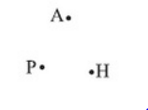### Question: 2

Draw a line in your notebook and name it using a small letter of the alphabet

### Solution:

Let us draw a line and name it l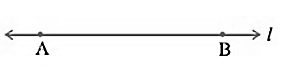### Question: 3

Draw a line in your notebook and name it by using two points on it

### Solution:

Let us first draw a line. Two points on it are P and Q. now, the line can be written as line PQ### Question: 4

Give three examples from your environment of:

i) Points

ii) Portion of a line

iii) Plane of a surface

iv) Portion of a plane

v) Curved surface

### Solution:

i) Points –

The period at the end of the sentence, a pinhole on the map and the point at which two walls and the floor meet at the corner of the room.

ii) Portion of a line –

Tightly stretched power cables, laser beams, and thin curtain rods

iii) Plane of a surface –

The surface of a smooth wall, the surface of the top of a table and the surface of a smooth white board

iv) Portion of a plane –

The surface of the sheet of the paper, the surface of calm water in a swimming pool and the surface of a mirror

v) Curved surface –

The surface of a gas cylinder, the surface of a tea pot and the surface of an ink pot.

### Question: 5

There are a number of ways by which we can visualize a portion of a line. State whether the following represent a portion of line or not:

i) A piece of elastic stretched to the breaking point

ii) Wire between two electric poles

iii) The line thread by which a spider lowers itself

i)  Yes

ii)  No

iii) Yes

### Question: 6

Can you draw a line on the surface of a sphere which lies wholly on it?

### Solution:

No, we cannot draw a line on the surface of the sphere, which lies wholly on it.

### Question: 7

Make appoint on the sheet of a paper and draw a line passing through it. How many lines can you draw through this point?

### Solution:

Unlimited number of lines can be drawn passing through a point L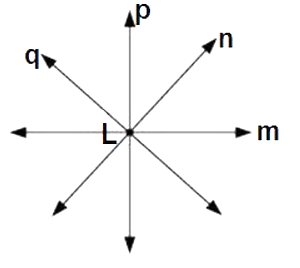### Question: 8

Mark any two points P and Q in your notebook and draw a line passing through the points.

How many lines can you draw passing through this both points?

### Solution:

We have two points P and Q and we draw a line passing through these two points.

Only one line can be drawn passing through these two points.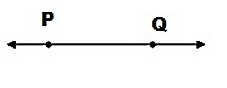### Question: 9

Give an example of the horizontal plane and a vertical plane from your environment.

### Solution:

Ceiling of a room is an example of a horizontal plane in our environment.

Wall of a room is an example of a vertical plane in our environment.

### Question: 10

How many lines may pass through one given point, two given point, any three collinear points?

### Solution:

Lines passing through one point – unlimited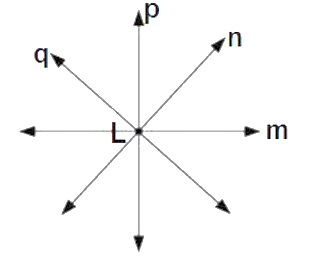Lines passing through two points – one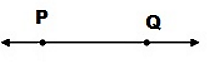Lines passing through any three collinear points – one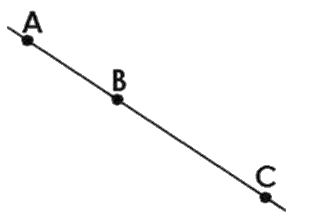### Question: 11

Is it ever possible for exactly one line to pass through three points?

### Solution:

Yes, it is possible if three points lie on a straight line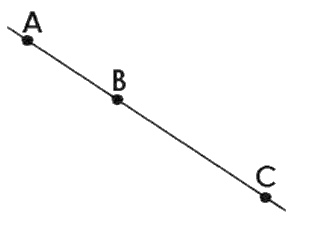### Question: 12

Explain why is not possible for a line to have a mid point?

### Solution:

The length of the line is infinite. Thus, it is not possible to find its midpoint. On the other hand, we can find, we can find the midpoint of a line segment

### Question: 13

Mark three non – collinear points points A, B, C in your notebook. Draw the lines through the points taking two at a time. Name these lines. How many such different lines can be drawn?

### Solution:

These are three non – collinear points A, B, C

Three lines can be drawn through these points. These three lines are AB, BC and AC### Question: 14

Coplanar points are the points that are in the same plane. Thus,

i) Can 150 points be coplanar?

ii) Can 3 points be non – co planar?

### Solution:

i) Yes,

A group of points that lie in the same plane are called co planar points.

Thus, it is possible that 150 points can be co-planar.

ii) No

Three points will be coplanar because we can have a plane that can contain 3 points on it.

Thus, it is not possible that 3 points will be non – coplanar.

### Question: 15

Using a ruler, check whether the following points given in the figure are collinear or not?### Solution:

i) D, A and C are collinear points

ii) A, B and C are non – collinear points

iii) A, B and E are collinear points

iv) B, C and E are non – collinear points

### Question: 16

Lines p, q is coplanar. So are the lines p, r. Can we conclude that the lines p, q, r are coplanar?

### Solution:

No, p, q and r are not necessarily coplanar.

Example – If we take p as intersecting line of two consecutive walls of a room, q as a line on the first wall and r on the second wall whose (both walls) intersection is line p

Thus we can see that p, q and r are not coplanar.

### Question: 17

Give three examples of each:

i) Intersecting lines:

ii) Parallel lines from your environment:

### Solution:

i) Intersecting lines: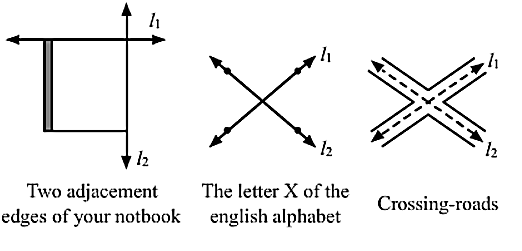ii) Parallel lines from your environment: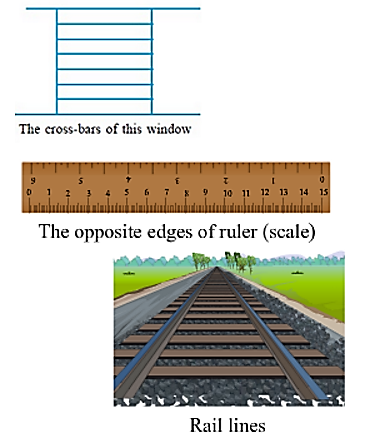### Question: 18

From the figure write:### Solution:

i) All pairs of intersecting lines – (l, m), (m, n) and (l, n)

ii) All pairs of intersecting lines: (l, p), (m, p), (n, p), (l, r), (m, r), (n, r), (I, q), (m, q),(n, q), (q, p), (q, r)

iii) Lines whose point of intersection is l: (m, p)

iv) Lines whose point of intersection is D: (l, r)

v) Lines whose point of intersection E: (m, r)

vi) Lines whose point of intersection is A: (l, q)

vii) Collinear points: (G, A, B and C) ,(D, E, J and F), ( G, H, I and J, K), (A, H and D), (B, I and E) and (C, F and K)

### Question: 19

Write concurrent lines and their and their point of concurrence: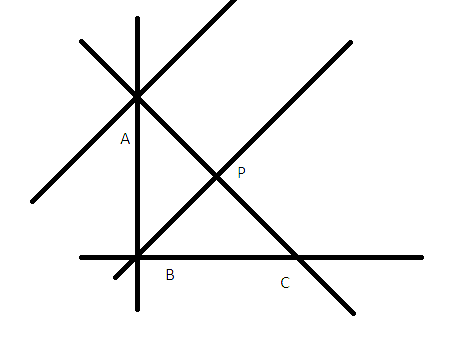### Solution:

From the given figure, we have:

Concurrent lines can be defined as three or more lines which share the same meeting point. Clearly lines, n, q, and l are concurrent with A as the point of concurrence.

Lines, m, q and p are concurrent with B as the point of concurrence.

### Question: 20

Mark four points A, B, C, D in your notebook such that no three of them are collinear. Draw all the lines which join them in pairs as shown### Solution:

i) How many such lines can be drawn

Six lines can be drawn through these four points as given in the figure.

ii) Write the names of these lines

These lines are AB, BC, CD, BD and AD

iii) Name the lines which are concurrent to A

Lines which are concurrent at A are AC, AB and AD

### Question: 21

What is the maximum number of points of intersection of three lines in a plane? What is the minimum number?

### Solution:

Maximum number of points of intersection of three lines in a plane will be threeMinimum number of points of intersection of three lines in a plane will be zero### Question: 22

With the help of a figure, find the maximum and minimum number of points of intersection of four lines in a plane.

### Solution:

Maximum number of points of intersection of four lines in a plane will be six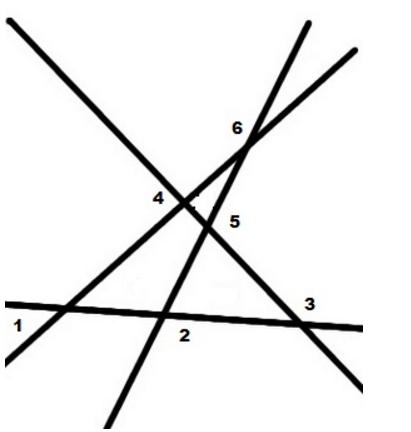Minimum number of points of intersection of four lines in a plane will be zero.### Question: 23

Lines p, q and r are concurrent. Also, the lines p, r and s are concurrent. Draw a figure and state whether lines p, q, r and s are concurrent or not?

### Solution: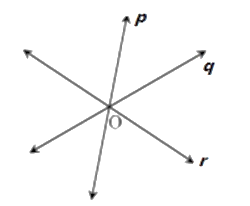Thus, lines p, q and r intersect at a common point O

Also, lines p, r and s are concurrent

Therefore, lines p, r, and s intersect at a common point. But q and r intersect each other at O.

So, p, q and r intersect at O

Hence, p, q, r and s are concurrent. Lines p, q, r and s intersect at O.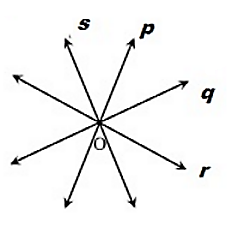### Question: 24

Lines p, q, and r are concurrent. Also lines p, s and t are concurrent. Is it always true that the lines q, r and s will be concurrent? Is it always true for lines q, r, and t?

### Solution:

Lines p, q, and r are concurrent. So, lines p, q and r intersect at a common point O.Given lines p, s, and t are concurrent. So, lines p, s and t also intersect at a common point. However, it is not always true that q, r and s or q, r and t are concurrent.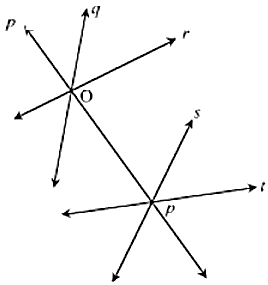### Question: 25

Fill in the blanks in the following statements using suitable words:

i) A page of a book is a physical example of a _____.

ii) An inkpot has both _______surfaces.

iii) Two lines in a plane are either ______ or are ________.

### Solution:

i) A page of a book is a physical example of a plane

ii) An inkpot has both curved and plane surfaces

iii) Two lines in a plane are either parallel or are intersecting

### Question: 26

State which of the following statements are true and which are false:

i) Point has a size because we can see it as a thick dot on paper

ii) By lines in geometry, we mean only straight lines

iii) Two lines in a plane always intersect at a point

iv) Any plane through a vertical line is vertical

v) Any plane through a horizontal line is horizontal

vi) There cannot be a horizontal line in a vertical plane

vii) All lines in a horizontal plane are horizontal

viii) Two lines in a plane always intersect at a plane

ix) If two lines intersect at a point P, then P is called the point of concurrence of the two lines

x) If two lines intersect at a point P, then P is called the point of intersection of the two lines

xi) If A, B, C and D are collinear points D, P and Q are collinear, then points A, B, C, D, P and Q are always collinear

xii) Two different lines can be drawn passing through two given points

xiii) Through a given point only one line can be drawn

xiv) Four points are collinear if any three of them lie on them lie on the same line

xv) The maximum number of points of intersection of three lines is three

xvi) The minimum number of points of intersection of three lines is one

i) False

ii) True

iii)  False

iv) True

v)  False

vi)  False

vii) True

viii)  False

ix) False

x) True

xi) False

xii)  False

xiii)  False

xiv) False

xv) True

xvi)  False

### Question: 27

Give the correct matching of the statements of column A and column B

 Column A Column B i Points are collinear a. May be parallel or intersecting ii Line is completely known b. Undefined terms in geometry iii Two lines in a plane c. If they lie on the same line iv Relations between points and lines d. Can pass through a point v Three non-collinear points e. Determine a plane vi A plane extends f. Are called incidence properties vii Indefinite number of lines g. If two points are given viii Point, line and plane are h Indefinitely in all directions

### Solution:

 Column A Column B i Points are collinear c. If they lie on the same line ii Line is completely known g. If two points are given iii Two lines in a plane a. May be parallel or intersecting iv Relations between points and lines f. Are called incidence properties v Three non-collinear points e. Determine a plane vi A plane extends h. Indefinitely in all directions vii Indefinite number of lines d. Can pass through a point viii Point, line and plane are b. Undefined terms in geometry## Complete JEE Main/Advanced Course and Test Series

OFFERED PRICE: Rs. 15,900
View Details Xpress Buy

### Course Features

• 731 Video Lectures
• Revision Notes
• Test paper with Video Solution
• Mind Map
• Study Planner
• NCERT Solutions
• Discussion Forum
• Previous Year Papers

### Course Features

• 728 Video Lectures
• Revision Notes
• Previous Year Papers
• Mind Map
• Study Planner
• NCERT Solutions
• Discussion Forum
• Test paper with Video Solution